Reach Us+44-1522-440391
On the Fundamental Theorem in Arithmetic Progression of Primes | OMICS International
Journal of Generalized Lie Theory and Applications
All submissions of the EM system will be redirected to Online Manuscript Submission System. Authors are requested to submit articles directly to Online Manuscript Submission System of respective journal.

# On the Fundamental Theorem in Arithmetic Progression of Primes

Jiang CX*

Institute for Basic Research, Palm Harbor, P. O. Box 3924, Beijing 100854, P. R. China

*Corresponding Author:
Jiang CX
Institute for Basic Research
Palm Harbor, P. O. Box 3924
Beijing 100854, P. R. China
Tel: +1-727-688 3992
E-mail: [email protected]

Received Date: March 10, 2017; Accepted Date: April 17, 2017; Published Date: April 27, 2017

Citation: Jiang CX (2017) On the Fundamental Theorem in Arithmetic Progression of Primes. J Generalized Lie Theory Appl 11: 264. doi: 10.4172/1736-4337.1000264

Copyright: © 2017 Jiang CX. This is an open-access article distributed under the terms of the Creative Commons Attribution License, which permits unrestricted use, distribution, and reproduction in any medium, provided the original author and source are credited.

Visit for more related articles at Journal of Generalized Lie Theory and Applications

#### Abstract

Using Jiang function we prove the fundamental theorem in arithmetic progression of primes. The primes contain only k<Pg+1 long arithmetic progression, but the primes have no k>Pg+1 long arithmetic progressions theorem.

#### Keywords

Arithmetic; Lie theory; Fundamental theorem; Progression; Asymptotic formula

#### Theorem

The fundamental theorem in arithmetic progression of primes.

We define the arithmetic progression of primes [1-3].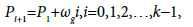(1)

Where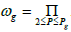is called a common difference, Pg is called g-th prime.

We have Jiang function [1-3]: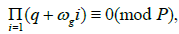(2)

X(P) denotes the number of solutions for the following congruence: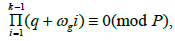(3)

Where q=1,2,…,P−1.

If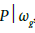then X(P)=0;X(P)=k−1 otherwise. From eqn (3) we have: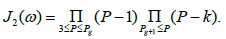(4)

If k=Pg+1 then J2(Pg+1)=0, J2(ω)=0, there exist finite primes P1 such that P2,…,Pk are primes. If k<Pg+1 then J2()≠0, there exist infinitely many primes P1 such that P2,…,Pk are primes. The primes contain only k<Pg+1 long arithmetic progression, but the primes have no k>Pg+1 long arithmetic progression geometry. We have the best asymptotic formula [1-3]: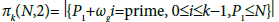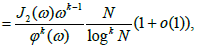(5)

Where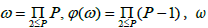is called primorial, φ(ω) Euler function.

Suppose k=Pg+1−1. From eqn (1) we have:

Pi+1=P1gi,i=0,1,2,…,Pg+12. (6)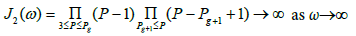(7)

We prove that there exist infinitely many primes P1 such that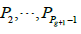are primes for all Pg+1.

From eqn (5) we have: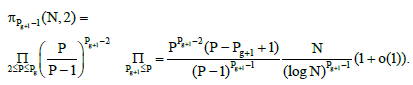(8)

From eqn (8) we are able to find the smallest solutions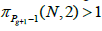for large Pg+1.

Theorem is foundation for arithmetic progression of primes.

Example 1: Suppose P1=2, ω1=2, P2=3. From eqn (6) we have the twin primes theorem:

P2=P1+2. (9)

From eqn (7) we have: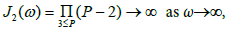(10)

We prove that there exist infinitely many primes P1 such that P2 are primes. From eqn (8) we have the best asymptotic formula [4-6]: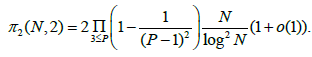(11)

Twin prime theorem is the first theorem in arithmetic progression of primes.

Example 2: Suppose P2=3, ω2=6, P3=5. From eqn (6) we have: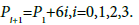(12)

From eqn (7) we have: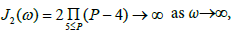(13)

We prove that there exist infinite many primes P1 such that P2, P3 and P4 are primes. From eqn (8) we have the best generalized asymptotic formula: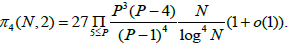(14)

Example 3: Suppose P9=23, ω9=223092870, P10=29. From eqn (6) we have: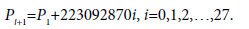(15)

From eqn (7) we have: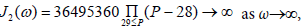(16)

We prove that there exist infinitely many primes P1 such that P2,…, P28 are primes. From eqn (8) we have the best asymptotic formula :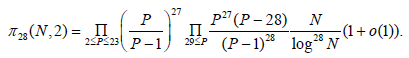(17)

From eqn (17) we are able to find the smallest solutions π28(N0,2)>1.

On May 17, 2008, Wroblewski and Raanan Chermoni found the first known case of 25 primes:

61711054912832631+366384×ω23×n, for n=0 to 24.

Theorem can help in finding for 26, 27, 28,…, primes in arithmetic progressions of primes.

Corollary 1: Arithmetic progression with two prime variables.

Suppose ωg=d. From eqn (1) we have:

P1,P2=P1+d,P3=P1+2d,…,Pk=P1+(k−1)d, (P1,d)=1 (18)

From eqn (18) we obtain the arithmetic progression with two prime variables: P1 and P2,

P3=2P2−P1, Pj=(j−1)P2−(j−2)P1, 3 ≤ j ≤ k < Pg+1. (19)

We have Jiang function :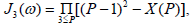(20)

X(P) denotes the number of solutions for the following congruence matrices: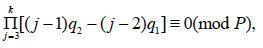(21)

where q1=1,2,…,P−1; q2=1,2,…,P−1.

From eqn (21) we have: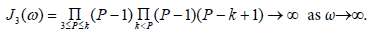(22)

We prove that there exist infinitely many primes P1 and P2 such that P3,…, Pk are primes for 3≤k<Pg+1.

We have the best asymptotic formula :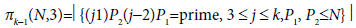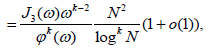(23)

From eqn (23) we have the best asymptotic formula: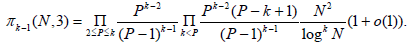(24)

From eqn (24) we are able to find the smallest solution πk−1(N0,3)>1 for large k<Pg+1.

Example 4. Suppose k=3 and Pg+1 >3. From eqn (19) we have:

P3=2P2−P1. (25)

From eqn (22) we have: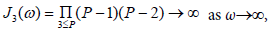(26)

We prove that there exist infinitely many primes P1 and P2 such that P3 are primes. From eqn (24) we have the best asymptotic formula: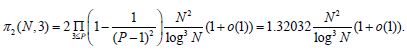(27)

Example 5: Suppose k=4 and Pg+1 >4. From eqn (19) we have:

P3=2P2−P1, P4=3P2−2P1. (28)

From eqn (22) we have: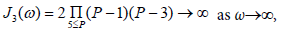(29)

We prove that there exist infinitely many primes P1 and P2 such that P3 and P4 are primes. From eqn (24) we have the best asymptotic formula: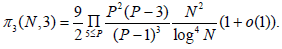(31)

Example 6: Suppose k=5 and Pg+1 >5. From eqn (19) we have:

P3=2P2−P1, P4=3P2−2P1, P5=4P2−3P1. (31)

From eqn (22) we have: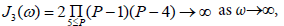(32)

We prove that there exist infinitely many primes P1 and P2 such that P3, P4 and P5 are primes. From eqn (24) we have the best asymptotic formula: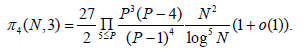(33)

Corollary 2: Arithmetic progression with three prime variables.

From eqn (18) we obtain the arithmetic progression with three prime Lie Theory variables: P1, P2 and P3.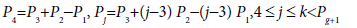(34)

We have Jiang function: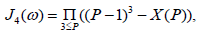(35)

X(P)denotes the number of solutions for the following congruence: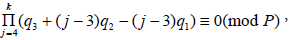(36)

Where qi=1,2,…,P−1, i=1,2,3.

Example 7: Suppose k=4 and Pg+1>4. From eqn (34) we have:

P4=P3+P2−P1. (37)

From eqns (35) and (36) we have: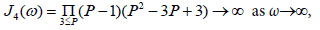(38)

We prove that there exist infinitely many primes P1 and P2 and P3 such that P4 are primes. We have the best asymptotic formula: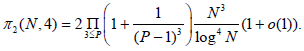(39)

For k≥5 from eqns (35) and (36) we have Jiang function: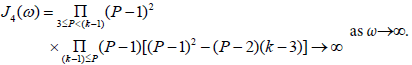(40)

We prove that there exist infinitely many primes P1 and P2 and P3 such that P4,…, Pk are primes for 5 ≤ k≤Pg+1.

We have the best asymptotic formula: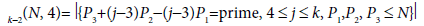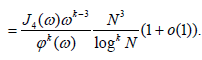(41)

From eqn (41) we have: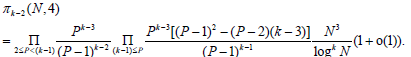(42)

From eqn (42) we are able to find the smallest solution πk−2(N0,4)>1 for large k<Pg+1.

Corollary 3: Arithmetic progression with four prime variables.

From eqn (18) we obtain the arithmetic progression of algebra with four prime variables: P1, P2, P3 and P4

P5=P4+2P3−3P2+P1, Pj=P4+(j−3)P3−(j−2)P2+P1, 5 ≤ j ≤ k < Pg+1 (43)

We have Jiang function: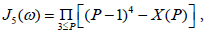(44)

X(P) denotes the number of solutions for the following congruence: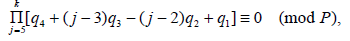(45)

Where,

qi=1,…,P−1,i=1,2,3,4

Example 8: Suppose k=5 and k<Pg+1>5. From eqn (43) we have:

P5=P4+2P3−3P2+P1. (46)

From eqns (44) and (45) we have: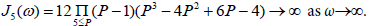(47)

We prove there exist infinitely many primes P1, P2, P3 and P4 such that P5 are primes.

We have the best asymptotic formula: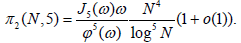(48)

Example 9: Suppose k=6 and Pg+1 >6. From eqn (43) we have:

P5=P4+2P3−3P2+P1, P6=P4+3P3−4P2+P1. (49)

From eqns (44) and (45) we have: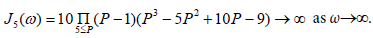(50)

We prove there exist infinitely many primes P1, P2, P3 and P4 such that P5 and P6 are primes.

We have the best asymptotic formula: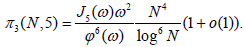(50)

For k≥7 from eqns (44) and (45) we have Jiang function: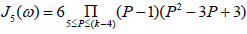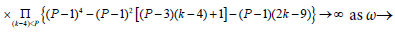(51)

We prove there exist infinitely many primes P1, P2, P3 and P4 such that P5,…,Pk are primes.

We have best asymptotic formula: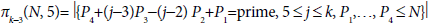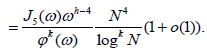(52)

#### Acknowledgements

I thank Professor Huang Yu-Zhen for computation of Jiang functions.

#### References

Select your language of interest to view the total content in your interested language

### Article Usage

• Total views: 1044
• [From(publication date):
April-2017 - Aug 19, 2019]
• Breakdown by view type
• HTML page views : 957
• PDF downloads : 87

## Post your commentCan't read the image? click here to refresh
###### Peer Reviewed Journals

Make the best use of Scientific Research and information from our 700 + peer reviewed, Open Access Journals

International Conferences 2019-20

Meet Inspiring Speakers and Experts at our 3000+ Global Annual Meetings

Top